# How to create custom user defined functions in Excel

For many tasks, regular Excel functions cannot help. We'll show you how to create new custom functions and how to use them to make your work easier. Learn how to create and use custom functions:

By the moment I started writing this article, Excel has already introduced you to over 450 different functions. With their help you can perform a vast variety of different operations. However, not any task can be solved in Excel as its developers couldn't foresee all the issues we face. I think that many of you have met at least one of these challenges:

• Not all the data can be processed by standard functions (for example, dates before 1900).
• Formulas can be quite long and complex. They are impossible to remember, difficult to understand, and hard to change to meet new criteria.
• Not all the tasks can be solved using standard Excel functions (for example, you cannot extract URL from a hyperlink).
• It is impossible to automate frequently repeated standard operations (such as importing data from an accounting program into an Excel sheet, formatting dates and numbers, removing unnecessary columns).

How can these problems be solved?

• Some users create an archive of workbooks with examples. They copy the desired formula from there and apply it to their spreadsheet.
• There are users who prefer creating a set of VBA macros once and run them whenever is needed.
• Another way to go is to create user defined functions (further — UDF) using the VBA editor.

While the first two options sound familiar, the third one may cause some confusion. So let’s have a closer look at custom functions in Excel and decide whether they are worth a shot.

## What is user defined function (UDF) in Excel?

UDF is a custom function that takes data, performs a calculation, and returns the desired result. The source data can be numbers, text, dates, booleans, and even arrays. The result of calculations can be a value of any type that Excel works with or an array of such values.

In other words, UDF is kind of an upgrade of standard Excel functions. You may use it when the capabilities of regular functions are not enough. Its main purpose is to supplement and expand the capabilities of Excel and perform actions that are impossible using standard functions.

There are several ways to create custom functions:

• Using Visual Basic for Applications (VBA). This method is described in this article.
• Using the great LAMBDA function that was introduced in Office 365.
• Using Office scripts. Currently, the scripts are available in Excel on the Web only.

Check out the screenshot below to see the difference between two ways of number extraction - using formula and a custom ExtractNumber() function.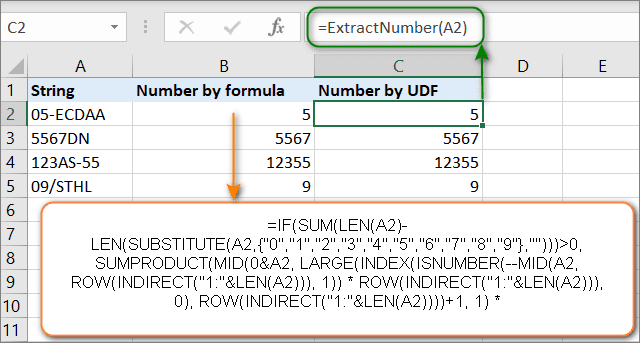You can use UDF in any of the following ways:

• As a formula, where it can take raw data from your worksheet and return a calculated value or an array of values.
• Like part of a VBA macro or other custom function code.
• As a component of your conditional formatting formulas.
• For storing constants and lists of data.

## How to create a custom function in Excel?

First of all, you need to open the Visual Basic Editor (VBE). Please keep in mind that it just opens in a new window and does not close your Excel spreadsheet.

The easiest way to open VBE is by using a keyboard shortcut - Alt + F11. It's fast, simple and there is no need to customize the Ribbon or Quick Access Toolbar.

Tip. Press Alt + F11 when VBE is open to go back to the Excel window.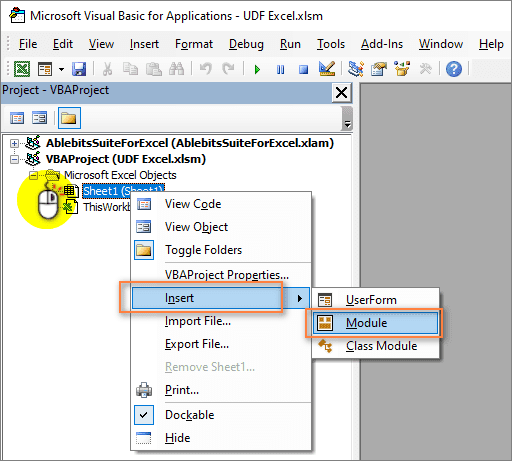After opening VBE, you need to add a new module where you will write your functions. Right-click on the VBA project pane and select Insert -> Module. An empty module window will appear where you are to specify your custom function.

Before we start, let's go through the rules by which UDFs are created:

• A user defined function always begins with “Function” and ends with “End Function”.
• “Function” is followed by the name of the function. This is a title you create and give to your function so that you could identify and use it later. This name must not contain spaces. If you want to separate words, use underscores. For example, Count_Words.
• Also, the name also cannot be the same as the names of standard Excel functions. If you do this, then the standard function will always be executed.
• The name of the user defined function cannot match the addresses of the cells in the worksheet. For example, the name ABC1234 is invalid.

Tip. It is highly recommended to give the functions descriptive names. Then you can easily select them from a long list of functions. For example, the name CountWords makes it easy to understand what the function does and apply it for word counting when needed.

• Next, the arguments of the function are usually listed in parentheses. This is the data with which it will work. There can be one or several arguments. If you have multiple arguments, you need to list them separated by commas.
• If the functions inside the UDF do not use arguments (for example, NOW, TODAY, or RAND), then you can create a function with no arguments. Also, no arguments are needed if you are using a UDF to store constants (such as pi).
• After that, specify the variables that the UDF uses. The type of these variables is indicated - number, date, text, array.
• Then you put several VBA statements that perform calculations using the arguments passed to the function.
• At the end, you should write a statement that assigns the final value to a variable with the same name as the function’s. This value gets returned to the formula from which the user defined function was called.
• Custom function code can include comments. They will help you remember the purpose of a function and its operators. If you want to make any changes in the future, the comments will be very helpful.

Note. A comment always starts with an apostrophe ('). The apostrophe tells Excel to ignore everything after it and until the end of the line.

Now let's try creating your first custom formula. For starters, we create a custom function that will count the number of words in a range of cells. To do this, insert this code into the module window: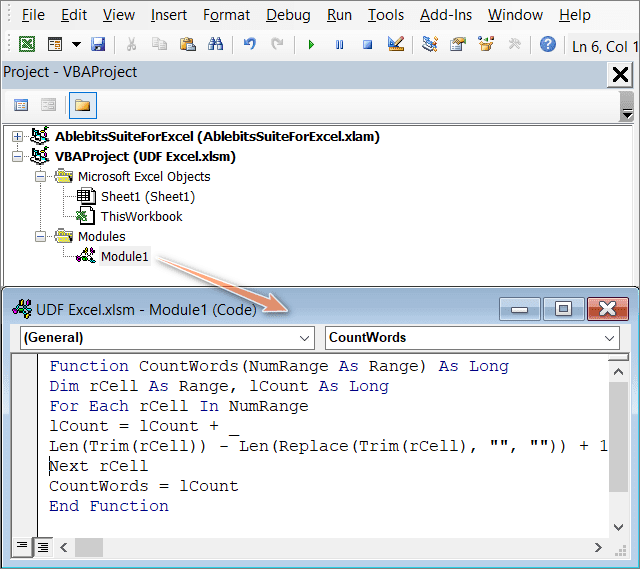I think some clarification may be needed here. As you remember from the rules above, UDF code always starts with a word “Function”. Then we make a description of the new function and close our UDF with “End Function”.

As we indicate in parentheses the initial data it will use, NumRange As Range means that the UDF argument will be a range of values. This function needs to return only one argument - the range of cells.

In the second line of code, we are declaring variables.

As Long indicates that the result of the CountWords function will be an integer.

The Dim statement declares two variables of our function:

• rCell is the variable of the range of cells in which we will count words.
• lCount is an integer variable that will contain the number of words.

The For Each argument is designed to perform calculations on each item in a group of items (range of cells). This loop operator is used when the number of elements in the group is unknown. We start with the first element, then we take the next one and so on to repeat until the very last value. The loop repeats as many times as there are cells in the input range.

Inside this loop, an operation that calculates the number of words is applied to the value of each and every cell:

Len (Trim(rCell)) - Len(Replace(Trim(rCell), " ", "")) + 1

As you can see, this is a regular Excel formula that uses the standard text functions: LEN, TRIM and REPLACE. Instead of the cell reference, we use the range variable rCell. Hence, for each cell of the range, we sequentially count the number of words in it.

The counted numbers are summed up and stored in the lCount variable:

`lCount = lCount + Len (Trim(rCell)) - Len(Replace(Trim(rCell), " ", "")) + 1`

When the loop is finished, the value of the variable is assigned to the function.

`CountWords = lCount`

The function returns the result of this variable to the cell of the worksheet, which is the total number of words.

It is this line of code that ensures that the function will return the lCount value to the cell from which it was called.

As you can see, it is not very difficult. Save your function by clicking the “Save” button on the VBE ribbon. After that, you can close the editor window. To do this, you can use the keyboard shortcut Alt + Q. Or just go back to the Excel sheet by pressing Alt + F11.

You can compare working with the custom function CountWords and counting the number of words using formulas and choose a method that fits you more.

## How to use custom functions

When you create a custom function, it becomes available in the same way as other standard Excel functions. Now we are going to learn how to create custom formulas.

To apply a user defined function, you have two options.

• Click the fx button on the formula bar. Among the categories of functions, you will see a new group - User Defined. And in this category, you can see our new custom function CountWords.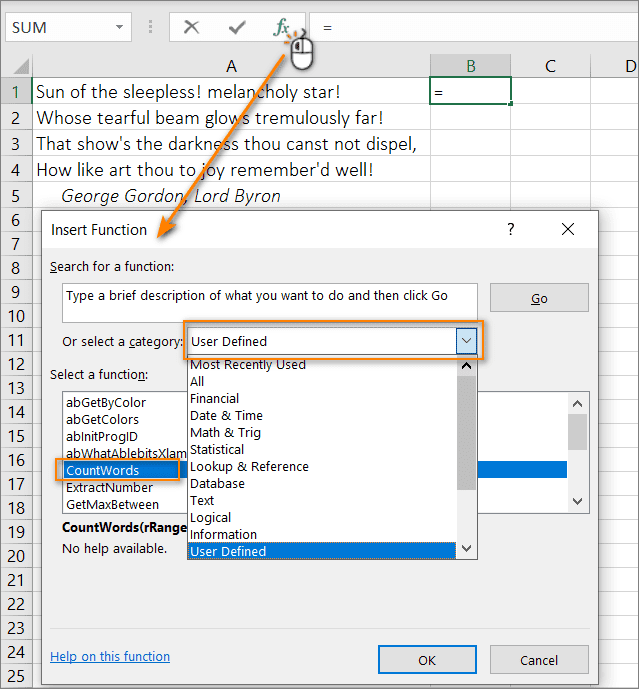• You can simply write this function into a cell in the same way as you do with standard functions. When you start typing a name, Excel will show you the name of the user defined function in a list of matching functions. In the example below, when I entered =cou, Excel showed me a list of matching functions, among which you see CountWords.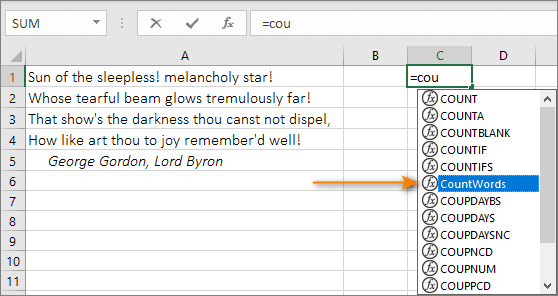Let’s finally apply our custom formula. Write it down in any cell:

`= CountWords(A1: A4)`

Press Enter. See, we just specified the function and set up a range and here is the result of the count: 28 words.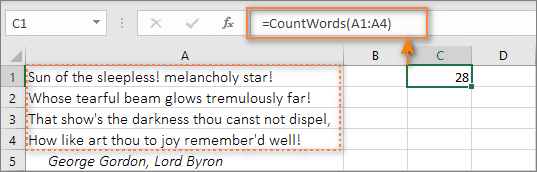## Various kinds of user defined functions

Now we’ll check different UDF types depending on the arguments they use and the results they return.

### No arguments

Excel has several standard functions that do not require arguments (RAND, TODAY, NOW). For example, the RAND function returns a random number between 0 and 1. The TODAY function will return the current date. You don't need to specify any values ​​to them.

Good news is that you can create such a function in VBA as well. Below is the code that will write the name of your worksheet into a cell:

Or you can use this code instead:

Notice that there are no arguments in the parentheses after the function name. Since the result to be returned does not depend on any values ​​in your working file, the function does not require any arguments.

The above code defines the result of the function as a string data type (since the desired result is a filename, which is text). If you do not specify the data type, then Excel will determine it on its own.

### With one argument

Now let's create a simple function that works with one argument which is one cell.

Our task is to extract the last word from the text string. Here is the code we’ll use:

The_Text is the value of the selected cell. We indicate that this should be a text value (As String).

The StrReverse function returns text in reverse character order. Next, the InStr function determines the position of the first space. Using the Left function, we get all characters ending with the first space. Then we remove the spaces with Trim. Change the order of characters again using StrReverse. We get the last word from the text.

Since this function takes a cell value, we don't need to use Application.Volatile here. As soon as the argument changes, the function will automatically update.

### Using an array as an argument

Many Excel functions use arrays of values ​​as arguments. Remember the SUM, SUMIF, SUMPRODUCT functions. We already covered this situation above when we learned how to create a custom function to count the number of words in a range of cells.

The code below creates a function that sums all even numbers in a specified range of cells.

The NumRange argument is specified as Range. This means that the function will use the original data array. It should be noted that the Variant variable type can also be used. It looks like

The Variant type provides a "non-dimensional" container for storing data. Such a variable can store any of the data types allowed in VBA, including numeric values, text, dates, and arrays. Moreover, the same such variable in the same program at different times can store data of different types. Excel will determine on its own what data is passed to the function.

The code has a For Each argument. It takes each cell and checks to see if it contains a number. If it is not, then nothing happens and it moves on to the next cell. If a number is found, it checks if it is even or not (using the MOD function).

All even numbers are summed up in the Result variable.

When the loop is finished, the Result value is assigned to the SumEven variable and passed to the function.

### With multiple arguments

Most Excel functions have multiple arguments. Custom functions are no exception. This is why it is so important to be able to create UDFs with multiple arguments.

The code below creates a function that selects the maximum number in a given range:

It has 3 arguments: a range of values, a lower bound for a numeric range, and an upper bound for a range. The first one is rngCells As Range. This is the range of cells to search for the maximum value in. The second and third arguments (MinNum, MaxNum) are specified without a type declaration. This means that the Variant data type will be applied to them by default.

VBA uses 6 different numeric data types. Specifying only one of them means limiting the use of the function. Therefore, it would be better if Excel determines the type of numeric data itself.

The For Each loops through all the values ​​in the selected range sequentially. The numbers that are in the range from the maximum to the minimum value are written to a special array - arrNums. Using the standard MAX function, find the largest number in this array.

### With required and optional arguments

To understand what an optional argument is, remember the VLOOKUP function. Its fourth argument [range_lookup] is optional. If you omit one of the required arguments, your function will throw an error. But if you omit the optional argument, your function will work.

However, optional arguments are not useless. They allow you to choose your calculation option.

For example, in the VLOOKUP function, if you omit the fourth argument, an approximate search will be performed. If you specify the [range_lookup] argument as FALSE (or 0), an exact match will be found.

If your user defined function has at least one required argument, it must be written at the beginning. Only then there are the optional ones.

To make an argument optional, you just need to add "Optional" before it. Let's see an example of a function with optional arguments in VBA:

This custom function retrieves text from a cell. Optional CaseText = False means the CaseText argument is optional. By default, its value is set to FALSE.

If the optional CaseText argument is TRUE, then the result is returned in uppercase. If the optional argument is FALSE or omitted, the result remains as-is, without changing the case.

You may wonder: "Can there be only optional arguments in a user-defined function?". We have an answer for you down below :)

### With only optional arguments

As far as I know, there is no built-in Excel function that only has optional arguments. Things may have changed from the day I wrote this article, but so far such a function doesn’t exist.

Anyways, it is possible to create a UDF with only optional arguments. As a proof, here is a custom function that writes a username to a cell:

As you can see, there is only one Uppercase argument, and it is optional.

If the argument is FALSE or omitted, then the username is returned unchanged. If the function argument is TRUE, the name is returned in uppercase characters (using the Ucase VBA function). Notice the first statement of the function. It contains the IsMissing VBA function, which detects the presence of an argument. If there is no argument, the statement sets the Uppercase variable to FALSE.

Have a look at another version of this function:

In this case, the optional argument defaults to FALSE. If the function is entered without arguments, then FALSE will be used by default and the username will be obtained without changing the case. If any value other than zero is entered, then all characters will be converted to uppercase.

### The return value is an array

VBA has a very useful function called Array. It returns a variant data type, which is an array (simply put, multiple values). If you are not familiar with array formulas in Excel, we suggest starting with the beginner’s guide to array functions on our blog.

UDFs that return an array are very useful when storing arrays of values. For example, the Months () function will return an array of month names:

Note. The UDF outputs data horizontally (in a row).

And what if you need a vertical array of values? It was mentioned before that UDFs can be used in Excel formulas along with standard functions. Having said that, let’s use Months() as an argument in the TRANSPOSE function:

`= TRANSPOSE(Months())`You can use UDFs to quickly enter data into a table as shown in the screenshot above. For example, in a sales report, you do not need to manually write the names of the months.

What is more, you can get the name of the month by its number. For example, cell A1 contains the number of the month. Then the name of the month can be obtained using the formula

`= INDEX (Months(), 1, A1)`

An alternative version of this formula:

`=INDEX({"January", "February", "March", "April", "May", "June "," July "," August "," September "," October "," November "," December "}, 1, A1)`

Agree, UDF with an array makes an Excel formula much easier.

If I managed to convince you that this function is worth trying, I recommend that you pay attention to the articles below.

## You may also be interested in

1. I am unable to use the excel engineering function complex( ) in an excel user-defined function. For a function named Tryit, I have tried the following:
tryit = complex(1,1) ' highlights "complex" and states "Compile error sub or function not defined"
tryit = WorksheetFunction.Complex(1,1) 'compiles and I can find it in the excel worksheet under UDF's. but when I execute it, I get "#Value"

How can I use complex(), improduct, imdiv, imsum, etc. in a UDF?

• Hi! Use the WorksheetFunction object to call the Excel function. For example, if you want to use the SUM function, you can write:

Dim result As Double
result = WorksheetFunction.Sum(Range("A1:A10"))

2. Len (Trim(rCell)) - Len(Replace(Trim(rCell), "", "")) + 1

doesn't work.

You need to use the substitute function instead of replace.

• Hi! Thank you for your comment! There is a space accidentally missing in the formula: lCount = lCount + Len(Trim(rCell)) - Len(Replace(Trim(rCell), " ", "")) + 1
We will fix this typo. If you are interested in the function of counting words in a cell, I recommend you to pay attention to this article: How to count words in Excel - formula examples.

3. I know it's 9 years later, but this is EXACTLY what I needed for my dreams of color coded accounting to become a reality! Thank you!

4. I have created a UDF with multiple arguments which is used on a specific worksheet and it is running perfectly. Whenever I do some unrelated calculations on another worksheet of the same workbook, the UDF on the previous worksheet throws #value error in place of the former correct return value. What might be the reason for this?

• Hi!
Perhaps in your UDF you are using data from "current worksheet". When you do calculations on another worksheet, it becomes new current worksheet.

5. Thank you for the UDF to count cells by color. It works perfectly, except... when a cell in the defined range changes color and should not be counted, the function does not refresh and the Data Refresh does not update the count either. I can copy/paste the function and it updates the count but this is not ideal. Is there a way to make this function update as cell colors change?

• Hi!
Unfortunately, changing the cell format in Excel does not recalculate formulas and custom functions.

• Im trying to use this, ive followed the instructions to add the UDF but its still not working. Is there anything common that i may have missed?

6. Hello,

I am new to this, but can I write a function where if a certain range of numbers are identified (I.e., 25-74) then a specific text response is generated?
Ie if (25-74) then “performance is in the average score range.”

I would like to do this for quite a number of cells.

7. Hello
I am on a deadline and need to know how to format dates formatted with a decimal to a 6-digit date. For example, I have 6500-row customer datasheet I'm working with. Many of the birthdates have been entered like this (03.28.1980) or (3.28.80) and 03/28/1980 and many other random ways. When I select "format cells" and chose the 03/28/80 - U.S. date format, only the dates with slashes or hyphens convert. The ones with a decimal point don't change and there are too many to convert manually. I am new to excel any help would be greatly appreciated.

• Hello!
If the dates are written as text in different ways, you cannot use the formula for all of them. We have a tool that can solve your task in a couple of clicks - Text to date tool. It is available as a part of our Ultimate Suite for Excel that you can install in a trial mode and check how it works for free.

• Hello,
Have you tried using the find and replace feature in Excel?
Maybe using it to replace all the decimal points with slashes will help the program recognize the cells as dates.
Since find and replace applies the changes to the whole sheet, it would be best to copy the dates away to a blank throwaway worksheet, do the changes there, then copy it all back.

• Not sure if this helps you a year later, but I had a similar problem. Let's say mixed days are in column C. First, I formatted all as dates. Then, I added a column D with =datevalue(C1) formula and stretched it all over down. This saved me a lot of time.

HOWEVER it is super important to review such data - dates like April 12th (where day < 13) might be handled unexpectedly as Excel might not know which one is the month.

8. Hello
thank you for the post,
I have created a function, but as I close the spreadsheet, it disappears..
How can I make it available to all new spreadsheets?
Thanks

9. I'm looking for a way to define UDFs within a namespace.
I need to call it in the following way
=Somenamespace.MyUDF() in the Excel, but vba doesn't allow namespace.
I know there is a way to do it in new javascript adding, but I need in to be done in vba module.
Is there someway to get around it?

10. Thank you for this article. It is a very good introduction, but tt wasn't exactly what I was hoping for.

I have been creating User Defined Functions (UDFs) of my own for quite a while now. What I am looking for is information on how to add the UDF's context information to the "Insert Function" dialog window. My UDF shows up there, but I do not know of a way of filling in the missing "Insert Function" UDF and Argument/Parameter information like the built in functions provided by Microsoft.